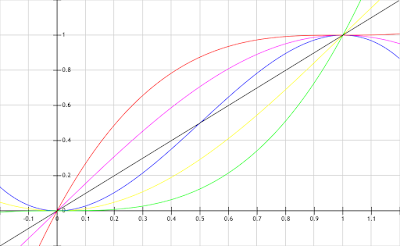### Ease functions

Ease functions are important for interpolation of just about anything. Popular functions are "smoothstep" or "ease in" and "ease out". This images shows what they look like for x[0,1] and y[0,1]:linear: y=x, ease out: y=(x-1)^3+1, ease in: y=x^3, smoothstep: y=3x^2-2x^3, y=sin(x*PI/2), y=1-cos(x*PI/2)
There are other popular functions for ease in and ease out, e.g. y=sin(x*PI/2) resp. y=1-cos(x*PI/2). What is important about those functions is if they're C1- and/or C2-continuous when you want to combine functions. C1-continuity means that the first derivative or the tangent of the function is the same for x=0 and x=1, meaning you can piece together two of the curves and there are no sudden changes or jumps for y. C2-continuity means that the second derivative or how the tangent changes is the same.
y=sin(x*PI/2) and y=1-cos(x*PI/2) are not C1-continuous, but you can use them to start/end a linear interpolation for example.

The nice picture was plotted with http://fooplot.com